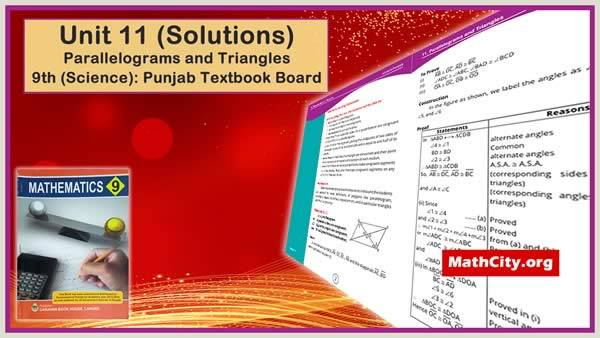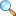# Unit 11: Parallelograms and Triangles

On this page notes of Unit 11 of Mathematics 9 written by Dr. Karamat H. Dar and Prof. Irfan-ul-Haq are given.After studying this unit, the students will be able to:

• prove that in a parallelogram
• the opposite sides are congruent,
• the opposite angles are congruent,
• the diagonals bisect each other.
• prove that if two opposite sides of a quadrilateral are congruent and parallel, it is a parallelogram.
• prove that the line segment, joining the midpoints of two sides of a triangle, is parallel to the third side and is equal to one half of its length.
• prove that the medians of a triangle are concurrent and their point of concurrency is the point of trisection of each median.
• prove that if three or more parallel lines make congruent segments on a transversal, they also intercept congruent segments on any other line that cuts them.

Quadrilateral: A figure formed by four non-collinear points in the plane is called a quadrilateral.

Parallelogram: A figure formed by four non-collinear points in the plane is called a parallelogram if its opposite sides are parallel.

Rectangle: A figure formed in the plane by four non-collinear points is called a rectangle if
(i) its opposite sides are equal in length.
(ii) the angle at each vertex is of measure 90 degree.

• Exercise 11.1 |View Online | Download PDF• Exercise 11.2 |View Online | Download PDF• Exercise 11.3 | Added soon
• Exercise 11.4 | Added soon
• Exercise 11.5 | Added soon
• Review Exercise |

Please click on View Online to see inside the PDF.

• matric/9th_science/unit11